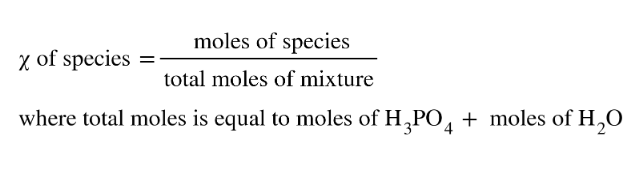# Problem: What are the mole fractions of H3PO4 and water in a solution of 14.5 g of H3PO4 in 125 g of water?(a) Outline the steps necessary to answer the question.(b) Answer the question.

###### FREE Expert Solution
• Recall that mole fraction (χ) can be calculated as:• Start calculating the moles of H3PO4 and H2O using the mass provided and their molar masses
• Then plugin these moles to the mole fraction equation
93% (310 ratings)###### Problem Details

What are the mole fractions of H3PO4 and water in a solution of 14.5 g of H3PO4 in 125 g of water?

(a) Outline the steps necessary to answer the question.